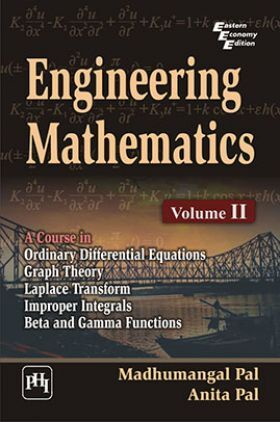# Engineering Mathematics : Volume 2

( 51 )
Sold ( 57 times )
18831 Views
MRP : ₹295.00
Price : ₹210.19
You will save : ₹84.81 after 29% Discount
Inclusive of all taxes
INSTANT delivery: Read it now on your device

Save extra with 2 Offers

Get ₹ 50

Instant Cashback on the purchase of ₹ 400 or above

Product Specifications

 Publisher PHI Learning All Engineering Mathematics books by PHI Learning ISBN 9788120339675 Author: PAL, MADHUMANGAL ,PAL, ANITA Number of Pages 416 Available Available in all digital devicesAbout The Book Engineering Mathematics

Book Summary:

This Volume II of Engineering Mathematics is a sequel to Volume I of Engineering Mathematics written by the same authors. It is designed for the second-semester course for undergraduate students of all branches of engineering. The text presents a thorough study of some mathematical techniques and exemplifies their use in solving problems encountered in various engineering courses.

The major part of the book is devoted to explaining the most important standard methods (including the Laplace transform method) available for solving ordinary differential equations. Such equations are of fundamental importance in mathematics because various real-life physical and geometrical problems lead to solving differential equations. Graphs, too, are the powerful tools in solving engineering problems. This book provides a sound introduction to the wide area of graph theory which is of paramount importance today in solving optimization problems. The final chapter of the book deals with the first and second type improper integrals including the Gamma and Beta functions.

KEY FEATURES :

Presents a clear and step-by-step presentation of the theory to aid the learning process.

Includes a large number of solved examples.

Chapter-end exercises provide multiple-choice questions and review questions.

Incorporates WBUT examination questions with their answers.

Preface
1. BASIC CONCEPTS OF DIFFERENTIAL EQUATIONS
2. DIFFERENTIAL EQUATIONS OF FIRST ORDER
3. DIFFERENTIAL EQUATIONS OF FIRST ORDER AND HIGHER DEGREE
4. LINEAR DIFFERENTIAL EQUATIONS OF SECOND AND HIGHER ORDER
5. SIMULTANEOUS LINEAR DIFFERENTIAL EQUATIONS WITH CONSTANT COEFFICIENTS
6. GRAPHS
7. PATHS, CIRCUITS AND CONNECTIVITY OF GRAPHS
8. TREES
9. MATRIX REPRESENTATION OF GRAPH
10. GRAPH THEORETIC ALGORITHMS
11. LAPLACE TRANSFORMS
12. INVERSE LAPLACE TRANSFORMS
13. SOLUTION OF ORDINARY DIFFERENTIAL EQUATIONS USING LAPLACE TRANSFORM
14. IMPROPER INTEGRAL, GAMMA AND BETA FUNCTIONS
University Question Papers (20022010)
Bibliography Index